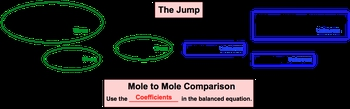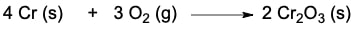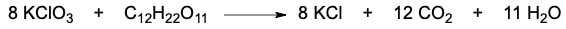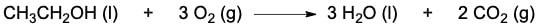Start typing, then use the up and down aroows to select an option from the list.

# GOB Chemistry

Learn the toughest concepts covered in your GOB - General, Organic, and Biological Chemistry class with step-by-step video tutorials and practice problems.

Chemical Reactions & Quantities

Stoichiometry deals with the numerical relationship between compounds in a balanced chemical equation.

## Stoichiometry

1

### concept

Stoichiometry1m
Play a video:
0
2

### concept

Stoichiometry3m
Play a video:
0

Utilize the stoichiometric chart to do all your stoichiometric calculations.3

### example

Stoichiometry Example 15m
Play a video:
0
4
Problem

The oxidation of chromium solid is represented by the following equation:How many moles of chromium (III) oxide are produced when 34.69 g Cr reacts with excess oxygen gas?

5
Problem

The reaction of potassium chlorate and sucrose is given below:If 2.33 x 10-7 formula units of potassium chlorate are reacted, how many grams of carbon dioxide will be produced?

6
Problem

If the density of ethanol, CH3CH2OH, is 0.789 g/mL, how many milliliters of ethanol are needed to produce 4.8 g of H2O in the following reaction?